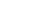# Digital Electronics Quiz Vol-1

This is Digital Electronics Quiz Vol -1 which is of the basic level to intermediate. Check your knowledge in Digital Electronics. After Completing this you can also move on to Digital Electronics Quiz Vol -2 which is more advanced.

1. How many flip-flops are there IC 7475?

2. AND gate is a logical gate that requires atleast______ input and produces only_______ outputs after performing the AND operation.

3. Select the correct logical expression for a NAND Gate from below

4. The Karnaugh map method of minimization of switching function is very convenient and effective if the number of variable in the switching function is

5. Which two of the following are universal gates?

6. Using the transformation method you can see that any POS realization of OR-AND with only _____

7. The Hexadecimal for binary 1001110 is

8. The three inputs AND gate has______number of operations.

9. A Karnaugh map (K-map) is an abstract form of ______ diagram organized as a matrix of squares.

10.Identify the Gate for this Symbol and Expression above

11. Logic Gates in digital electronics are

12. Which of the following expressions is in the sum-of-products (SOP) form?

13. One of De Morgan’s theorems states thatSimply stated, this means that logically there is no difference between:

14. An AND Gate of 3 inputs, 2 are high and one is low. What will be the output?

15. In Boolean algebra, the bar sign (—) indicates______ gate operation.

16. How many entries in a truth table are necessary for an AND Gate with 4 inputs______

17. 2’s complement of the binary number 10110 is

18. Which of the following gate will give a 0 when both of its inputs are 1?

19. The gate that can be used to implement any Boolean expression is ________

20. Decimal number 45 in binary is

21. IC Number of an AND gate is______

22. In a binary number system, the first digit (bit) from right to left is called______

23. A Logical gate whose output is low when the inputs are same and output is high when inputs are differ, is called_________

24. A latch is a _______ device in electronics circuits with 2 stable states.

25. The Hexadecimal of the decimal number 45.0 is ______

26. Applying DeMorgan’s theorem to the expression, we get ________

27. The base of the octal number system is

28. A three digit decimal number requires ________ for representation in the conventional BCD format.

29. 1’s complement of the binary 101101 is

30. The basic memory elements in digital circuits are made up of

31. A ______ is a universal gate which is an AND Gate followed by NOT gate.

32. A NOR Gate is an OR gate followed by a

33. Latch is a device with ___

34. The function of a NOT Gate is to

35. In an X-NOR Gate, if  input A is ‘0’ and input B is ‘1’, then the output will be

36. Convert the binary 111011 into decimal

Question 1 of 36